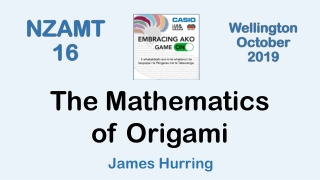DownloadDownload PresentationJames Hurring

# James Hurring

Télécharger la présentation## James Hurring

- - - - - - - - - - - - - - - - - - - - - - - - - - - E N D - - - - - - - - - - - - - - - - - - - - - - - - - - -
##### Presentation Transcript

1. The Mathematics of Origami James Hurring

2. Surds and A4 paper • What is the area of the kite? • What is the ratio of the diagonals of the kite? • What is the perimeter of the kite? • What is the ratio of the sides of the small rectangle?

3. Folding paper into Thirds and Fifths (Coordinate Geometry)

4. Folding a Hyperbolic Paraboloid (Complex roots of quadratics)

5. Folding Conic Sections

6. Folding Conic Sections

7. Angle Rules with a Triangle (Geometric Reasoning)

8. The Axioms of Folding

9. Folding a 60o Angle

10. Trisecting an Angle

11. Making a simple protractor

12. Doubling a cube Start by folding into thirds

13. Folding Pentagons (Geometric Reasoning)

14. 3-D Right-angled Triangles

15. Analysing the Crease pattern for Great Icosahedron (Non-right-angled Trigonometry)

16. Hamiltonian Graphs • Edge models of Polyhedra • (Colouring the edges)

17. Experimental Probability

18. Graphs & Patterns

19. Polyhedra Euler’s Rule Vertex Deficiency and 720o